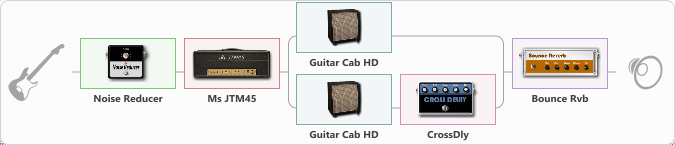# Simple Crunch

Discussion in 'ToneLib-GFX presets' started by era-z, Apr 20, 2022.

1. Simple Crunch
Preset name: Simple Crunch

Stratocaster bridge single (ssS)

Effects chain:Effect: "Noise Reducer" (Dynamics / Filter), active - "yes"
{

"Sens" = 70
"Mode" = Hard
}

Effect: "T-Scream" (Overdrive / Distortion), active - "yes"
{
"Drive" = 0
"Tone" = 50
"Level" = 80
}
Effect: "Ms JTM45" (Amp simulators), active - "yes"
{

"Gain" = 100
"Bass" = 30
"Middle" = 70
"Treble" = 100
"Presence" = 52
"Master" = 50
"Level (dB)" = 0
}

Effect: "Splitter" (Dynamics / Filter)
{

"A-Bypass" = Off
"A-Pan" = -40
"A-Level" = 55
"B-Bypass" = Off
"B-Pan" = 40
"B-Level" = 55
"Width" = 0
'A' branch:
{

Effect: "Guitar Cab HD" (Cabinets), active - "yes"
{

"Model" = Marshall 1960A (4x12")
"Mic Model" = Dynamic SM57
"Mic Position" = Center
"Mic Distance" = Near
"Low Cut (Hz)" = 60
"Hi Cut (kHz)" = 20.0
"Mix" = 100
"Level (dB)" = 0
}
}
'B' branch:
{

Effect: "Guitar Cab HD" (Cabinets), active - "yes"
{

"Model" = Peavey 5150 (4x12")
"Mic Model" = Ribbon M160
"Mic Position" = Center
"Mic Distance" = Near
"Low Cut (Hz)" = 80
"Hi Cut (kHz)" = 5.0
"Mix" = 100
"Level (dB)" = -1
}
Effect: "CrossDly" (Delay), active - "yes"
{

"Time" = 150
"Feedback" = 2
"Tone" = 40
"Sens" = 0
"Mix" = 68
}
}
}

Effect: "Bounce Rvb" (Reverberation), active - "yes"
{

"Time" = 5.0
"PreDelay" = 21
"LoDamp" = 36
"HiDamp" = 42
"Mix" = 25
}

Note: You will need to download and install the ToneLib-GFX software to use the preset.

#### Attached Files:

• ###### Simple_Crunch.tlgfx
File size:
751 bytes
Views:
2,221
Last edited: Apr 21, 2022
Elaman and kraggi like this.